var myUrl = 'https://www.irvine.science/2017/04/06/first-jupyteripython-notebook/'; if(window.top.location.href !== myUrl) { window.top.location.href = myUrl; } 
In [ ]:
# Analysis Script for Keys Online
🐝


## Setting up libraries and definitions¶

First we import the different Python libraries:

In :
%matplotlib inline
import os
import sys
import json
import re
import csv
import math
import pprint
import numpy as np
randn = np.random.randn
from numpy import nan
import pandas as pd
from pandas import *
import matplotlib.pyplot as plt
import matplotlib.patches as mpatches
import matplotlib
import scipy.stats as ss
from scipy import stats, integrate

import seaborn as sns
sns.set(color_codes=True)

np.random.seed(sum(map(ord, "distributions")))


## Input information & set up data storage¶

Input participant numbers and location of folders.

Invoke dictionary for data

In :
#Change this to whatever you need
# input_dir = "/Users/Alex/Documents/Javascript_truecolours/Keys_Orienting/parsed/"
input_dir = "/home/irvinea/Desktop/Javascript_truecolours/Keys_Orienting/parsed/"
partno = [38, 37, 36, 35, 34, 33, 32, 30, 29, 28, 27, 25, 24, 23, 21, 20, 19, 18, 17, 16, 15, 14]
# output_dir = "/Users/Alex/Documents/Javascript_truecolours/Keys_Orienting/results/"
output_dir = "/home/irvinea/Desktop/Javascript_truecolours/Keys_Orienting/results/"
# config_dir = "/Users/Alex/Documents/Javascript_truecolours/Keys_Orienting/conf/"
config_dir = "/home/irvinea/Desktop/Javascript_truecolours/Keys_Orienting/conf/"
#acknowledge directory
os.chdir(input_dir)
workingdirectory = input_dir

# This generates the empty dictionary in the desired nested format
data = {}
for part in partno:
data[part] ={}
for block in range(7):
data[part][block] = {}


## Read in data from CSV files¶

Each session is read in as a panda.DataFrame in to the data dictionary, this gives the following structure:

data[partno][blockno].field[trial]



So if I wanted to access participant 33, block 6 I would enter:

data



If I wanted just the image number in the first trial:

data.image
In :
#Now we read in the actual CSV files: if data is missing it will be recorded as NaN
for part in partno:
for block in range(10):

try:
#Reads the CSV file as a panda DataFrame into the Dict at the right location
data[part][block] = pd.read_csv(input_dir + str(part) + '_' + str(block+1) + '.csv')
#The parsing script sometimes makes empty files, with just headers. The line below checks if there is data in the read in CSV
data[part][block].rename(columns= lambda x: x.replace(" ", ""), inplace=True)
data[part][block]['image']
except:
#if an exception is raised, the file does not exist or is corrupted
data[part][block] = {nan}


## Display a summary of the data¶

We now need to return how complete each participants data is, we do this using the matplotlib

In :
#Make a dataframe: participants x 10 blocks, set to 0
summary_mat = [[0 for x in range(10)] for y in range(len(data))]

#Loop through matrix and insert #1 if data is present
mt_cnt = 0
for part in partno:
for block in range(10):
#see if this block has more than one row of trial data
try:
data[part][block][:1]
summary_mat[mt_cnt][block] = 1
except:
pass
mt_cnt +=1

#make the table
# define labels for columns and rows
columns = ('day 1', 'day 2', 'day 3', 'day 4', 'day 5', 'day 6', 'day 7', 'day 8', 'orienting', 'memory test')
rows = list(map(str, partno))
pads = matplotlib.figure.SubplotParams(left = 0.2, right = 1, bottom = 0.2, top = 1, wspace = 1, hspace = 1)
#plot out the bar chart
the_table = plt.bar(range(10),  list((np.array(summary_mat)).mean(axis=0)))

#Make map for cell colours in the table (green = complete, red = absent)
cellcolours = np.empty_like(summary_mat, dtype='object')# empty array for colours
for r in range(len(summary_mat)): # loop through rows
for c in range(len(summary_mat)): #loop through columns
if summary_mat[r][c] == 1: #if data exists
cellcolours[r,c] = 'g'
else:                      #else there is not data
cellcolours[r][c] = 'r'

#plot out the table
the_table = plt.table(cellText=summary_mat,colLabels=columns, rowLabels=rows, cellColours=cellcolours)

#print the plot
plt.show()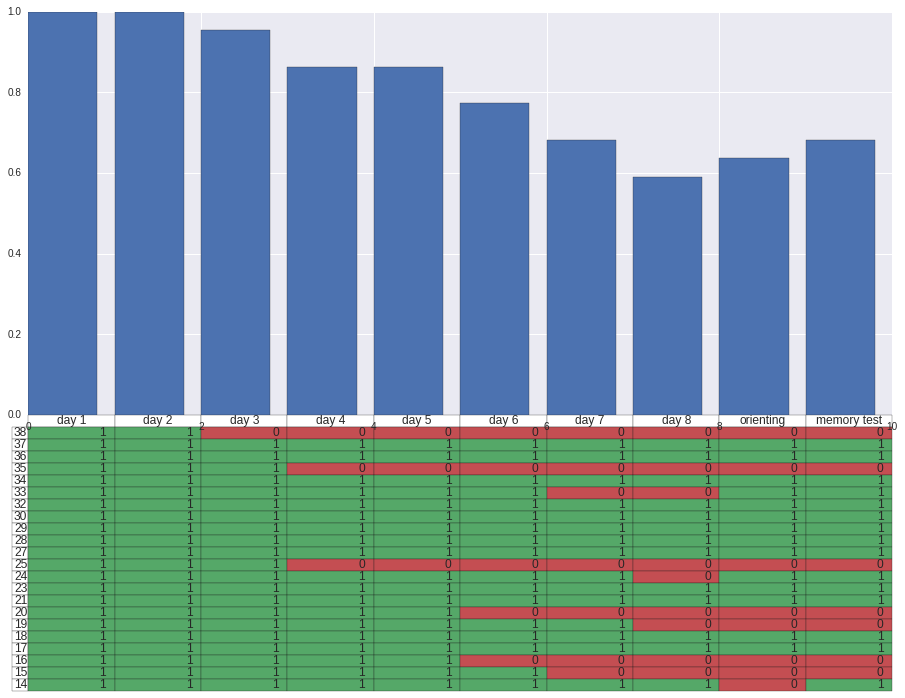## Look at the training data¶

Now let's look at how well the participants learnt each image in each order condition over the duration of training.

We want to still include the participants with missing days so NaN this

In :
data = pd.DataFrame(data)
rts = {}
rts_all = {}
#populate empty array
for day in range(8):
rts[day] = {}
rts_all[day] = {}
for order in range(4):
rts[day][order] = []
rts_all[day][order] = []

#print(data[part][day](data[part][day]['correct'] == 1) & (data[part][day]['order']==1))
#append rows to array - exception if non existent (i.e. missing days)
for part in partno:
for day in range(8):
try:
#only use correct trials
rts[day].append(np.array(data[part][day][(data[part][day]['order'] == 1) & (data[part][day]['correct'] == 1)]))
rts[day].append(np.array(data[part][day][(data[part][day]['order'] == 2) & (data[part][day]['correct'] == 1)]))
rts[day].append(np.array(data[part][day][(data[part][day]['order'] == 3) & (data[part][day]['correct'] == 1)]))
rts[day].append(np.array(data[part][day][(data[part][day]['order'] == 4) & (data[part][day]['correct'] == 1)]))

#use all trials
rts_all[day].append(np.array(data[part][day][data[part][day]['order'] == 1]))
rts_all[day].append(np.array(data[part][day][data[part][day]['order'] == 2]))
rts_all[day].append(np.array(data[part][day][data[part][day]['order'] == 3]))
rts_all[day].append(np.array(data[part][day][data[part][day]['order'] == 4]))
except:
pass

#concat vertically all data in cells for each dayXcondition
for day in range(8):
for order in range(4):
rts[day][order] = np.vstack(rts[day][order])
rts_all[day][order] = np.vstack(rts_all[day][order])


### RT Across Training¶

Graph for looking at RT across training

In :
#make an array of means and SD (day x condition)
means = np.zeros((8,4))
sd = np.zeros((8,4))
for day in range(8):
for order in range(4):
means[day, order] = np.mean(rts[day][order][:,5])#average RT
sd[day, order] = ss.sem(rts[day][order][:,5])#Standard Error of the Mean

#Plot the graph
rt_training = plt.figure(figsize=(15,9))
rt_training = plt.plot(range(len(means[:,0])), means[:,0], color = 'red')
rt_training = plt.fill_between(range(len(means[:,0])),means[:,0]-sd[:,0], means[:,0]+sd[:,0], alpha=0.5, color = 'red')
rt_training = plt.axvspan(6, 7, ymin=0, ymax=0.02, alpha = 0.5, color = 'red', zorder=0)
rt_training = plt.plot(means[:,1], color = 'blue')
rt_training = plt.fill_between(range(len(means[:,1])),means[:,1]-sd[:,1], means[:,1]+sd[:,1], alpha=0.5, color = 'blue')
rt_training = plt.axvspan(4, 5, ymin=0, ymax=0.02, alpha = 0.5, color = 'blue', zorder=0)
rt_training = plt.plot(means[:,2], color = 'green')
rt_training = plt.fill_between(range(len(means[:,2])),means[:,2]-sd[:,2], means[:,2]+sd[:,2], alpha=0.5, color = 'green')
rt_training = plt.axvspan(2, 3, ymin=0, ymax=0.02, alpha = 0.5, color = 'green', zorder=0)
rt_training = plt.plot(means[:,3], color = 'orange')
rt_training = plt.fill_between(range(len(means[:,3])),means[:,3]-sd[:,3], means[:,3]+sd[:,3], alpha=0.5, color = 'orange')
rt_training = plt.axvspan(0, 1, ymin=0, ymax=0.02, alpha = 0.5, color = 'orange', zorder=0)

#custom colour patches
red_patch = mpatches.Patch(color='red', label='AAAB', alpha=0.5)
blue_patch = mpatches.Patch(color='blue', label='AABA', alpha=0.5)
green_patch = mpatches.Patch(color='green', label='ABAA', alpha=0.5)
orange_patch = mpatches.Patch(color='orange', label='BAAA', alpha=0.5)

#Add legend to the plot: custom patches with manual text size
rt_training = plt.legend(handles=[red_patch, blue_patch, green_patch, orange_patch], prop={'size':16})

#Axis labels and plot title
rt_training = plt.ylabel('Reaction Time (ms)', fontsize=18)
rt_training = plt.xlabel('Training Day', fontsize=18)
rt_training = plt.suptitle('RT Across Days for Correct Trials', fontsize=18, fontweight='bold')
#show the plot
plt.show()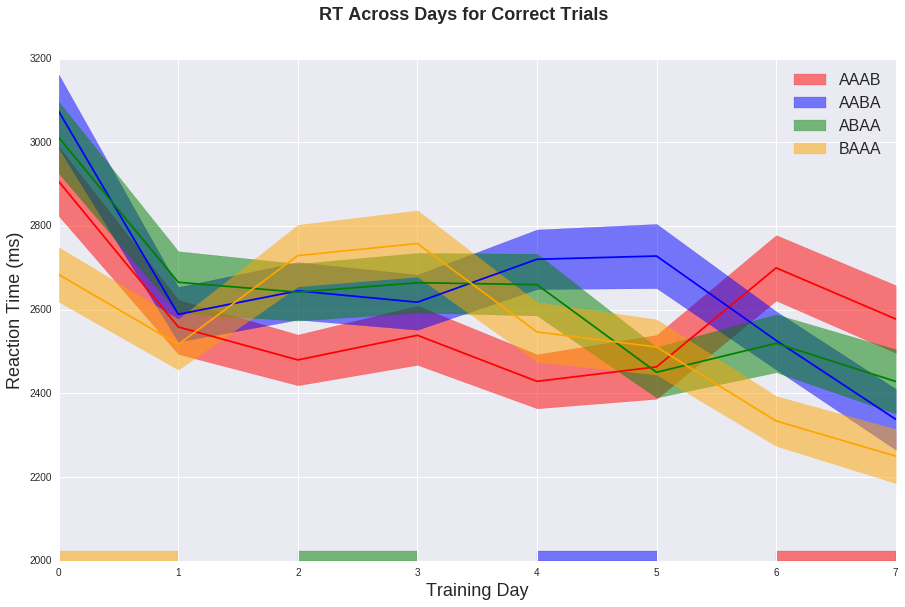### Accuracy Across Days¶

Graph for accuracy across training

In :
#make an array of means and SD (day x condition)
means = np.zeros((8,4))
sd = np.zeros((8,4))
for day in range(8):
for order in range(4):
means[day, order] = np.mean(rts_all[day][order][:,8])#average RT
sd[day, order] = ss.sem(rts_all[day][order][:,8])#Standard Error of the Mean

#Plot the graph
rt_training = plt.figure(figsize=(15,9))
rt_training = plt.plot(range(len(means[:,0])), means[:,0], color = 'red')
rt_training = plt.fill_between(range(len(means[:,0])),means[:,0]-sd[:,0], means[:,0]+sd[:,0], alpha=0.5, color = 'red')
rt_training = plt.axvspan(6, 7, ymin=0, ymax=0.02, alpha = 0.5, color = 'red', zorder=0)
rt_training = plt.plot(means[:,1], color = 'blue')
rt_training = plt.fill_between(range(len(means[:,1])),means[:,1]-sd[:,1], means[:,1]+sd[:,1], alpha=0.5, color = 'blue')
rt_training = plt.axvspan(4, 5, ymin=0, ymax=0.02, alpha = 0.5, color = 'blue', zorder=0)
rt_training = plt.plot(means[:,2], color = 'green')
rt_training = plt.fill_between(range(len(means[:,2])),means[:,2]-sd[:,2], means[:,2]+sd[:,2], alpha=0.5, color = 'green')
rt_training = plt.axvspan(2, 3, ymin=0, ymax=0.02, alpha = 0.5, color = 'green', zorder=0)
rt_training = plt.plot(means[:,3], color = 'orange')
rt_training = plt.fill_between(range(len(means[:,3])),means[:,3]-sd[:,3], means[:,3]+sd[:,3], alpha=0.5, color = 'orange')
rt_training = plt.axvspan(0, 1, ymin=0, ymax=0.02, alpha = 0.5, color = 'orange', zorder=0)

#custom colour patches
red_patch = mpatches.Patch(color='red', label='AAAB', alpha=0.5)
blue_patch = mpatches.Patch(color='blue', label='AABA', alpha=0.5)
green_patch = mpatches.Patch(color='green', label='ABAA', alpha=0.5)
orange_patch = mpatches.Patch(color='orange', label='BAAA', alpha=0.5)

#Add legend to the plot: custom patches with manual text size
rt_training = plt.legend(handles=[red_patch, blue_patch, green_patch, orange_patch], prop={'size':16})

#Axis labels and plot title
rt_training = plt.ylabel('Proportion Correct', fontsize=18)
rt_training = plt.xlabel('Training Day', fontsize=18)
rt_training = plt.suptitle('Accuracy Across Days', fontsize=18, fontweight='bold')
#show the plot
plt.show()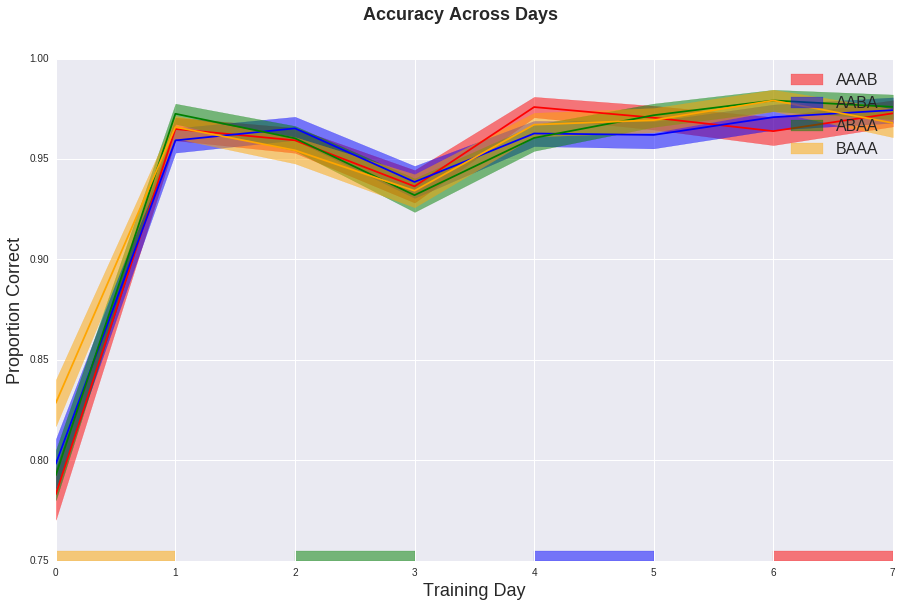## Orienting Results¶

Now let's look at the data from the orienting task.

First we are going to do some pretty non-pythonic messing around with data structure (I am still learning).

In :
#populate empty array - there was errors doing it in a more sensible way - first plotting script so lets see
orient= {}
all_orient= {}
for cond in range(2):
orient[cond]= {}
all_orient[cond]= {}
for order in range(4):
orient[cond][order]= []
all_orient[cond][order]= []

#append rows to array - exception if non existent (i.e. missing days)
for part in partno:
try:
#only use correct trials
orient.append(np.array(data[part][(data[part]['correctdirection'] == 1) & (data[part]['condition'] == 1) & (data[part]['order'] == 1)]))
orient.append(np.array(data[part][(data[part]['correctdirection'] == 1) & (data[part]['condition'] == 1) & (data[part]['order'] == 2)]))
orient.append(np.array(data[part][(data[part]['correctdirection'] == 1) & (data[part]['condition'] == 1) & (data[part]['order'] == 3)]))
orient.append(np.array(data[part][(data[part]['correctdirection'] == 1) & (data[part]['condition'] == 1) & (data[part]['order'] == 4)]))
orient.append(np.array(data[part][(data[part]['correctdirection'] == 1) & (data[part]['condition'] == 2) & (data[part]['order'] == 1)]))
orient.append(np.array(data[part][(data[part]['correctdirection'] == 1) & (data[part]['condition'] == 2) & (data[part]['order'] == 2)]))
orient.append(np.array(data[part][(data[part]['correctdirection'] == 1) & (data[part]['condition'] == 2) & (data[part]['order'] == 3)]))
orient.append(np.array(data[part][(data[part]['correctdirection'] == 1) & (data[part]['condition'] == 2) & (data[part]['order'] == 4)]))
#all trials
all_orient.append(np.array(data[part][(data[part]['condition'] == 1) & (data[part]['order'] == 1) & (data[part]['correctdirection'] != 9)]))
all_orient.append(np.array(data[part][(data[part]['condition'] == 1) & (data[part]['order'] == 2) & (data[part]['correctdirection'] != 9)]))
all_orient.append(np.array(data[part][(data[part]['condition'] == 1) & (data[part]['order'] == 3) & (data[part]['correctdirection'] != 9)]))
all_orient.append(np.array(data[part][(data[part]['condition'] == 1) & (data[part]['order'] == 4) & (data[part]['correctdirection'] != 9)]))
all_orient.append(np.array(data[part][(data[part]['condition'] == 2) & (data[part]['order'] == 1) & (data[part]['correctdirection'] != 9)]))
all_orient.append(np.array(data[part][(data[part]['condition'] == 2) & (data[part]['order'] == 2) & (data[part]['correctdirection'] != 9)]))
all_orient.append(np.array(data[part][(data[part]['condition'] == 2) & (data[part]['order'] == 3) & (data[part]['correctdirection'] != 9)]))
all_orient.append(np.array(data[part][(data[part]['condition'] == 2) & (data[part]['order'] == 4) & (data[part]['correctdirection'] != 9)]))
except:
pass

#concat vertically all data in cells for each dayXcondition
for cond in range(2):
for order in range(4):
orient[cond][order] = np.vstack(orient[cond][order])
all_orient[cond][order] = np.vstack(all_orient[cond][order])

# covert to DF because nested structure is actually a dict, which is not indexable by slices
orient = pd.DataFrame(orient)
all_orient = pd.DataFrame(all_orient)


### RT for conditions and order¶

This will be two plots - one with RT by condition for all order, and one for condition x order. MATPLOTLIB with subplots.

In :
#Takes Means and Standard Error.
#Due to the nested structure of orient (cond1[order1,2,3,4], cond2[order1,2,3,4]]) we have concat using np.vstack
cond_mean = np.array([np.mean(np.vstack(orient[:])[:,5]), np.mean(np.vstack(orient[:])[:,5])])
cond_error = np.array([np.std(np.vstack(orient[:])[:,5]), np.std(np.vstack(orient[:])[:,5])])

#make 2D array for this one. 2x4; condition x order
condxorder_mean = np.array([[np.mean(orient[:,5]), np.mean(orient[:,5]), np.mean(orient[:,5]), np.mean(orient[:,5])], [np.mean(orient[:,5]), np.mean(orient[:,5]), np.mean(orient[:,5]), np.mean(orient[:,5])]])
condxorder_error = np.array([[np.std(orient[:,5]), np.std(orient[:,5]), np.std(orient[:,5]), np.std(orient[:,5])], [np.std(orient[:,5]), np.std(orient[:,5]), np.std(orient[:,5]), np.std(orient[:,5])]])

f, rt_orient = plt.subplots(2, sharey=True, figsize=(15,9))

#First subplot - by condition
rt_orient.bar(range(len(cond_mean)), cond_mean*1000, 0.8, yerr = cond_error*1000)
rt_orient.set(ylabel="RT", xticks = np.arange(len(cond_mean)), xticklabels = ('75%', '25%'))

#second subplot - condition x order
color_scheme = ['red', 'blue', 'green', 'orange']
rt_orient.bar(range(len(condxorder_mean)), condxorder_mean*1000,
0.35, yerr = condxorder_error*1000, label = '75%', color = color_scheme)
rt_orient.bar(np.arange(len(condxorder_mean)) + 0.35, condxorder_mean*1000,
0.35, yerr = condxorder_error*1000, label = '25%', color = color_scheme, alpha =0.5)
plt.show()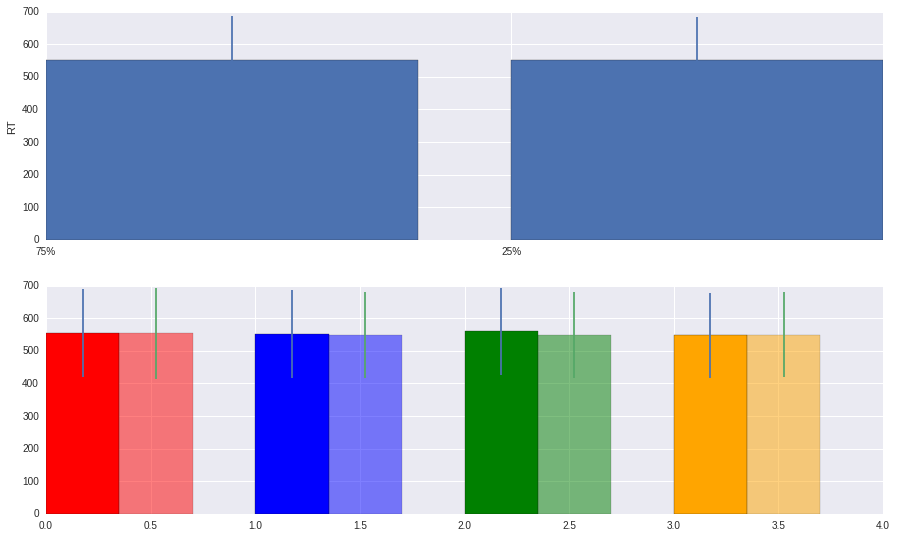#### Distribution of RTs¶

Using Seaborn let's quickly plot some distributions

In :
#RT
rt_cond = sns.kdeplot((np.vstack(orient[:])[:,5]), label = "75%") #75%
rt_cond = sns.kdeplot((np.vstack(orient[:])[:,5]), label = "25%") #25%
rt_cond.set(xlabel='RT (s)', ylabel = 'Frequency')
plt.show()

y, acc_cond = plt.subplots(2, sharex = True)
acc_cond = sns.countplot((np.vstack(all_orient[:])[:,12]), label = "75%") #75%
acc_cond = sns.countplot((np.vstack(all_orient[:])[:,12]), label = "25%") #25%
acc_cond.set(xlabel='Proportion correct', ylabel = 'Frequency')
plt.show()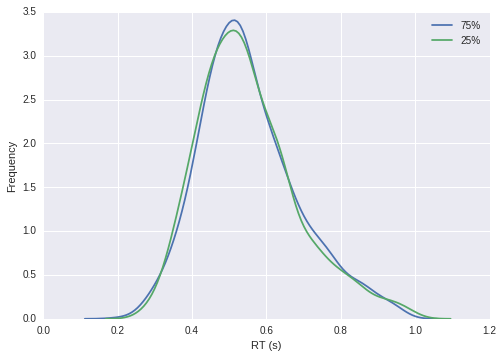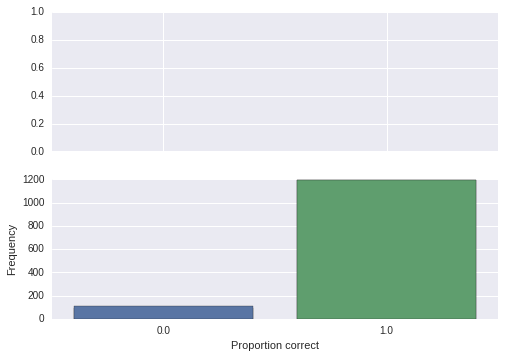## Memtest Results¶

Now let's look at memory accuracy, we can then look at differences in orienting rt between recall and non-recalled.

We can look at explicit recall accuracy by comparing euclidian distance between clicked location and actual location.

### Experimenting with concatenated data structure¶

Up until this point I have used a nested pandas DataFrame structure to organise the data, after doing this I think it might be more efficient to concatenate all of the data, and dummy code things like participant and day. So lets try this below:

In :
if 'concat_data' in locals():
del(concat_data) #do this because of the use of in locals() loop, which would not recognise a re-run

for part in partno:
for day in range(10):
try:
if 'concat_data' in locals():#does variable exist locally?
temp_data = np.hstack((np.array(data[part][day]), np.reshape([part]*len(data[part][day]),(len(data[part][day]), 1)), np.reshape([day]*len(data[part][day]),(len(data[part][day]), 1))))
concat_data=np.concatenate((concat_data, temp_data), axis=0)#concat
else:
concat_data=np.hstack((np.array(data[part][day]), np.reshape([part]*len(data[part][day]),(len(data[part][day]), 1)), np.reshape([day]*len(data[part][day]),(len(data[part][day]), 1))))
except: #if it doesnt work because participant X has no data for that day
pass

#Now we can easily subset data by any desired value 😊


### How accurate were clicks?¶

It looks like the original coordinates for the two conditions in each image are missing. So we need to load in the configuration information for each participant, from this we can work out the two locations for each image, and then compare them to the actual clicked location in the memtest

So let's load in this config information:

In :
if 'concat_conf' in locals():
del(concat_conf)

for part in partno: #loop through participants

with open(config_dir + str(part) + '.JSON') as data_file:    #open the file
data_temp = json.load(data_file)  #assign to temp variable

#We know there are 8 days in the training, each image switches at different days, so we must go through all days configurations
#However all days contain the order information for a given image number.......
#We can read this in from day1, then use this to find out which day the second coordinates are hidden in.
#loop for 192 images
for i in range(192):
#values for this image:
#for i in range(8)
img_no = data_temp["Key"]["day1"][i]
cond = data_temp["Key"]["day1"][i]
order = data_temp["Key"]["day1"][i]
if (order == 4):#if order is four, then the x,y coordinates given will be from the 25% condition
x_2 = data_temp["Key"]["day1"][i]
y_2 = data_temp["Key"]["day1"][i]
#we will need to get the 75% location from elsewhere (the irregular days)
#In this case we use day3, which will be the first day with new location!
#the i for i,x in enumerate(data_temp....).. finds the row number of the x,y for that image in day1
#we can not do a simple [==img_no] row number because it is a dictionary of lists rather than an array
x_1 = data_temp["Key"]["day4"][[i for i,x in enumerate(data_temp["Key"]["day4"]) if x == img_no]]
y_1 = data_temp["Key"]["day4"][[i for i,x in enumerate(data_temp["Key"]["day4"]) if x == img_no]]
else:#if order is not four, then the x,y coordinates given will be from the 75% condition
x_1 = data_temp["Key"]["day1"][i]
y_1 = data_temp["Key"]["day1"][i]
#to find out where the 25% coordinates are we will fetch this from order*2, this gives us the less commmon day
x_2 = data_temp["Key"]["day" + str(2*(5-order))][[i for i,x in enumerate(data_temp["Key"]["day" + str(order*2)]) if x == img_no]]
y_2 = data_temp["Key"]["day" + str(2*(5-order))][[i for i,x in enumerate(data_temp["Key"]["day" + str(order*2)]) if x == img_no]]

if 'concat_conf' in locals():#does variable exist locally?
concat_conf=np.vstack((concat_conf,np.array([img_no, cond, order, x_1, y_1, x_2, y_2, part])))
else:
concat_conf=np.array([img_no, cond, order, x_1, y_1, x_2, y_2, part])

del(data_temp)


Cartesian things: Okay, now we have this information (annoying it wasn't there) we can check whether the clicks in the memtest were accurate. We can do this using the Euclidean distance:

$Distance = \sqrt{(x_{2}-x_{1})^2 + (y_{2}-y_{1})^2}$

In Python:

distance_1 = math.sqrt((memtest_data[row, 2]-ref_info)**2  +  (memtest_data[row, 3]-ref_info)**2)

distance_2 = math.sqrt((memtest_data[row, 2]-ref_info)**2  +  (memtest_data[row, 3]-ref_info)**2)



We can also calculate this for each location that was clicked in the memtest. Comparing each click with both the possible locations, allows us to coarsely categorise the location it may have been refering too [WARNING: This may not be what they were doing, hence the word coarsely].

Coordinate conversion

Also we have to convert coordinates recorded from PyscoPy, where the center of the screen is (0px,0px) to JS, which are only in quadrant I of the cartesian plane, screen center is (500px,375px). This is because PsychoPy records clicks relative to the center, wheras Javascript records it relative to the image canvas.

We can do this by the converting function: $f(x_{JS},y_{JS}) = x_{memtest}+500, y_{memtest}+375$

Output

The script below takes all of this information and outputs an array with the accuracy of clicks in each participant's memtest results.

In :
if 'accur_array' in locals():
del(accu_array)
memtest_data = concat_data[(concat_data[:,14] == 9)] #select all memtest results
for row in range(len(memtest_data)): #loop through each memtest result
no = memtest_data[row,13] #participant of current row
image = memtest_data[row, 0] #image on current row
confid = memtest_data[row,9]
ref_info = concat_conf[(concat_conf[:, 7] == no) & (concat_conf[:, 0] == image)]

#Euclidean distances between click and both learnt locations of the key: with conversion to common coord system
eucl_1 = math.sqrt(((memtest_data[row, 2]+500)-ref_info)**2  +  ((memtest_data[row, 3]+375)-ref_info)**2)
eucl_2 = math.sqrt(((memtest_data[row, 2]+500)-ref_info)**2  +  ((memtest_data[row, 3]+375)-ref_info)**2)

#which one is best? Force to use on of the coordinates, flag which one (2=75%, 1=25%)
if (eucl_1 > eucl_2):
eucl = eucl_2
eucl2 = eucl_1
target = 2
else:
eucl = eucl_1
eucl2 = eucl_2
target = 1

if 'accu_array' in locals(): #if this is not the first loop and array exists
accu_array = np.vstack((accu_array, np.array([image, confid, eucl, eucl2, target, no])))
else: #if first loop create the array
accu_array = np.array([image, confid, eucl2, eucl, target, no])


### Confidence Accuracy?¶

OK, so let's look if the confidence ratings people gave for their two clicks per image have a statistically significant relationship?

This can be done with a simple one way ANOVA using scipy stats. Then plotted in matplotlib

In :
#accuracy of closest click means and error from each confidence rating
acc_means = (np.mean(accu_array[:,2][accu_array[:,1] == 1]), np.mean(accu_array[:,2][accu_array[:,1] == 2]), np.mean(accu_array[:,2][accu_array[:,1] == 3]))
degrees_free = len(np.unique(accu_array[:,5]))
acc_err = (ss.sem(accu_array[:,2][accu_array[:,1] == 1], ddof=degrees_free), ss.sem(accu_array[:,2][accu_array[:,1] == 2], ddof=degrees_free), ss.sem(accu_array[:,2][accu_array[:,1] == 3], ddof=degrees_free))

#locations and widths of bars
ind = np.arange(3)
width = 0.7

#make figure
conf_acc = plt.figure()
conf_acc = plt.bar(ind+width, acc_means, width, yerr=acc_err)

#custom labels
# add some text for labels, title and axes ticks
conf_acc= plt.ylabel('Accuracy in pixels')
conf_acc= plt.xlabel('Confidence')
conf_acc= plt.xticks(ind + width , ['1', '2', '3'])

plt.show()

#Are there differences in an one way ANOVA ?
ANOVA = ss.f_oneway(accu_array[:,2][accu_array[:,1] == 1], accu_array[:,2][accu_array[:,1] == 2], accu_array[:,2][accu_array[:,1] == 3])
print('ANOVA One Way, F = ', ANOVA , ' P-Value = ', "%.10f" %ANOVA)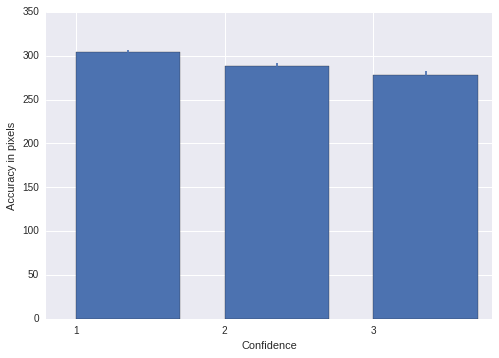('ANOVA One Way, F = ', 12.995975482751984, ' P-Value = ', '0.0000023368')


Great! There is a small (but significantly significant) association between confidence and accuracy. But there is still not a large magnitude difference at the group level. Let's see if it varies much between participants though.

In :
#Let's try seaborn for this task
# Draw a nested barplot grouping accuracy by confidence and participant
accu_conf_group = sns.barplot(x=list(map(int, accu_array[:,5])), y=accu_array[:,2], hue=list(map(int, accu_array[:,1])))
plt.show(accu_conf_group)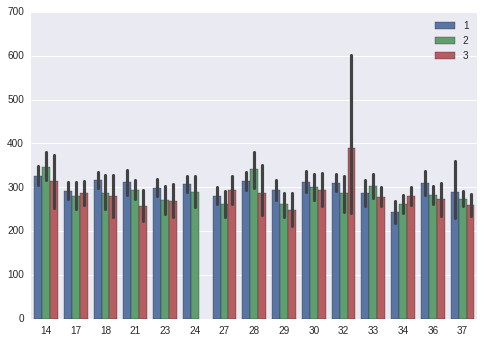## Orienting results grouped by accuracy¶

Now we have our accuracy results for each image, we can look at the orienting RT for target's participants remembered against ones that they did not.

In :
#Load in all orienting data from main array
orient_data = concat_data[(concat_data[:,14] == 8)]
#what distance do we want to set as a threshold in pixel distance?
thresh = 100
#empty arrays
rt_poor = []
rt_good = []
acc_poor = []
acc_good = []
for part in partno:
#Select all trials which match participant number, and are not invalid trials (9)
for trial in range(len(orient_data[(orient_data[:,13] == part) & (orient_data[:,12] != 9)])):
#temp orienting data to make things easier to follow
temp_orient = orient_data[(orient_data[:,13] == part) & (orient_data[:,12] !=  9)]
#accuracy data from memtest for this participant
temp_acc = accu_array[accu_array[:,5] == part]
#image number of this trial
img_no = temp_orient[trial, 0]
#accuracy information for this image, which matches the probed target location

#NOTE: this can be from 0-2 values, because sometimes BOTH clicked locations in the memory test are closer-
#to one of the target location. If the other target location was probed in orienting it is excluded, as bo-
#-th the participant's memory clicks were closer to the other location (that was not probed).
acc4trial = temp_acc[:,2][(temp_acc[:,4] == temp_orient[trial,6]) & (temp_acc[:,0] == img_no)]

#if no accuracy information exists for this row, do nothing
if len(acc4trial) == 0:
pass

#else if accuracy exists
else:
#if participant made a clear distinction in memtest results acc4trial will be one number so below will work
try:
#if the accuracy value was higher than threshold we categorise this as poor
if acc4trial > thresh:
#for RT only select correct trials
if temp_orient[trial,12] == 1:
rt_poor.append(temp_orient[trial,5])

acc_poor.append(temp_orient[trial,12])
#if the accuracy value was lower than threshold we categorise this as good
else:
if temp_orient[trial,12] == 1:
rt_good.append(temp_orient[trial,5])
acc_good.append(temp_orient[trial,12])

except:
pass

#if there was an error above then below will work, if no error it will just pass on
#We assume that there is two values, and we chose the best estimate, so this is a liberal measure
try:
#Choose the lowest number (i.e. with the highest accuracy)
if acc4trial > acc4trial:
acc4trial = acc4trial
else:
acc4trial = acc4trial
#as above categorise it is a poor or good
if acc4trial > thresh:
if temp_orient[trial,12] == 1:
rt_poor.append(temp_orient[trial,5])
acc_poor.append(temp_orient[trial,12])
else:
if temp_orient[trial,12] == 1:
rt_good.append(temp_orient[trial,5])
acc_good.append(temp_orient[trial,12])
except:
pass

#function for plot
def plot_per_acc(poor, good, ylab, title):
x = [1.5,2.5]
xlabels = ['poor', 'good']
y = [np.mean(poor), np.mean(good)]
yer = [ss.sem(poor), ss.sem(good)]

#Make the plot
orient_acc = plt.bar(x,y, yerr=yer)
orient_acc= plt.ylabel(ylab)
orient_acc= plt.xlabel('Accuracy Group')
orient_acc= plt.xticks(x, xlabels)
orient_acc = plt.title(title)
plt.show()

#Print stats
print('poor: ', np.mean(poor), 'good: ', np.mean(good))
print('SEM: ', ss.sem(poor), 'SEM: ', ss.sem(good))
print('no. poor: ', len(poor), 'no. good: ', len(good))
print('number of orienting trials with no associated nearest memtest click: ', 192*sum(np.array(summary_mat)[:,9]) -(len(poor)+len(good)))

plot_per_acc(acc_poor, acc_good, 'Accuracy', 'Proportion correct x Accuracy')
plot_per_acc(rt_poor, rt_good, 'RT (ms)', 'Proportion correct x RT')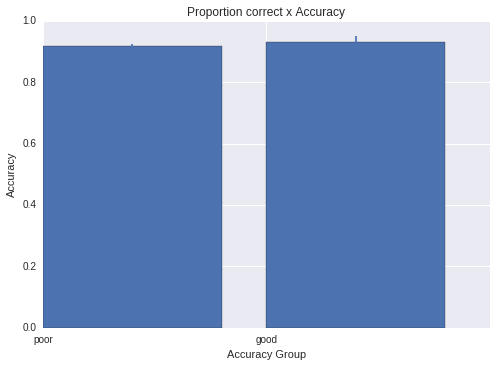('poor: ', 0.91774094536525475, 'good: ', 0.93269230769230771)
('SEM: ', 0.0068096487480690344, 'SEM: ', 0.017414715148125849)
('no. poor: ', 1629, 'no. good: ', 208)
('number of orienting trials with no associated nearest memtest click: ', 1043)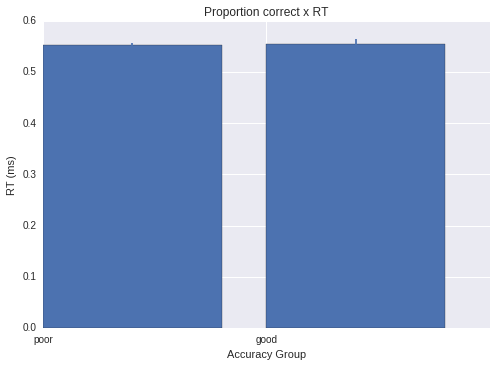('poor: ', 0.55340326014016727, 'good: ', 0.55504982397020097)
('SEM: ', 0.003503003846328112, 'SEM: ', 0.0097086767221823377)
('no. poor: ', 1495, 'no. good: ', 194)
('number of orienting trials with no associated nearest memtest click: ', 1191)